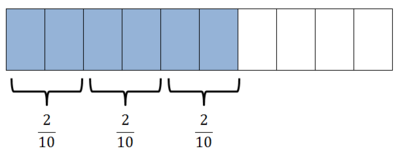# Dan’s Division Strategy

Alignments to Content Standards: 6.NS.A.1

Dan observes that $$\frac{6}{10}\div \frac{2}{10} = 6 \div 2$$ He says,

I think that if we are dividing a fraction by a fraction with the same denominator, then we can just divide the numerators.

Is Dan’s conjecture true for all fractions? Explain how you know.

## IM Commentary

The purpose of this task is to help students explore the meaning of fraction division and to connect it to what they know about whole-number division.

The Standards for Mathematical Practice focus on the nature of the learning experiences by attending to the thinking processes and habits of mind that students need to develop in order to attain a deep and flexible understanding of mathematics. Certain tasks lend themselves to the demonstration of specific practices by students. The practices that are observable during exploration of a task depend on how instruction unfolds in the classroom. While it is possible that tasks may be connected to several practices, only one practice connection will be discussed in depth. Possible secondary practice connections may be discussed but not in the same degree of detail.

This particular task helps illustrate Mathematical Practice 3, “Construct viable arguments.” Students are asked to analyze a division problem and ascertain whether the strategy used will continue to work for all similar division problems.  The student must explain their reasoning procedurally, conceptually or both.  The explanations allow students to verbalize why their strategy is effective and how fraction division connects to whole-number division. Their explanation solidifies their understanding of rational number operations by building on their prior knowledge. Their use of prior knowledge when constructing viable arguments leads into the second part of MP 3, critiquing the reasoning of others. A possible classroom demonstration might be a student explaining multiple fraction division problems similar to Dan’s and expressing to other students why Dan is correct by showing how the common denominator allows for the numerators to be divided as in whole number operations. The teacher can then start generating questions such as “Will this work if the denominators are different?  Why or why not?” This type of math talk in the classroom is built through collaborative problem solving and dialogue.

## Solutions

Solution: A procedural explanation and a conceptual one.

Yes, Dan's rule is correct for all fractions.

Explaining with analogies: One way of explaining why the rule is correct is to bear in mind that $6\over 10$ refers to six "items", where the "item" is $1\over 10$. $${6\over 10}=6\,\Bigl({1\over 10}\Bigr),$$ so the original division problem can be rephrased as $${6\,\Bigl({1\over 10}\Bigr)}\div{2\,\Bigl({1\over 10}\Bigr)}.$$ The computation $${6\,\Bigl({1\over 10}\Bigr)}\div{2\,\Bigl({1\over 10}\Bigr)} = 6\div 2 =3$$ follows the same logic as: $$6\,{\rm apples}\div 2\,{\rm apples} = 6\div 2 = 3.$$ We would probably not write it as $6\,{\rm apples}\div 2\,{\rm apples}$; instead we might have a situation where we have 6 apples and want to know how many groups of 2 apples we can make. Since we can make 3 groups with 2 apples in each group, the answer is 3. There was nothing special about the apples, the same reasoning applies to any "objects":

How many groups of 2 peaches can I make if I have 6 peaches?

How many groups of \$2 dollars can I make if I have \$6?

How many groups of 2 tenths can I make if I have 6 tenths?

When we divide a quantity consisting of $m$ units divided into groups of size $n$ of the same units, then the result does not depend on what the units are. The answer is found by dividing the number $m$ by the number $n$.

In summary, Dan's rule is true not only for dividing fractions with the same denominator, but also for any division of one quantity (number of units) by another quantity with the same units. In all cases, we find the answer by dividing the numbers, and the kind of unit does not matter.

Explaining with symbols: Dan might have made his conjecture based on using the "invert and multiply" rule: $${6\over 10}\div{2\over 10} = {6\over 10}\times{10\over 2}= {6\times 10\over 2\times10} = {6\over 2} = 6\div 2.$$ This works just as well for any denominator $d\not=0$. If $m$ and $n$ are any integers and $n\not=0$, then: $${m\over d}\div{n\over d} = {m\over d}\times{d\over n} = {m\times d\over n\times d} = {m\over n} = m\div n.$$

Submitted by J. Madden. This solution was developed by the 12 middle- and secondary-school teachers in the "LaMSTI On-Ramp Course" at LSU, with major contributions from N. Revaula.

Solution:

A diagram helps make this clear:There are 6 tenths shaded, and we want to know how many groups of 2 tenths we can make; there are 3 such groups. We can see that there is nothing special about the fact that the small rectangles represent tenths; the same reasoning would work for any denominator.